### Overview

# Top

At the core of the LifterLMS grading systems are quizzes and (with the LifterLMS Assignments Add-on) assignments. Quizzes and assignments are attached to lessons and are used to require additional steps to be taken before progressing a course forward, to test the student’s comprehension of lesson content, and much more.

The grades of a student’s quizzes and assignments serve as the base numbers used in all grading calculations.

By default, LifterLMS weights all quizzes and assignments equally but by leveraging weighting options available for lessons, quizzes, and assignments it’s easy to place greater emphasis on selected lessons, quizzes, or assignments.

This document pertains specifically to grading configuration options and how grading works, to learn how to grade a student’s quizzes or assignments see our guides: How to Grade and Review Quizzes or Grading and Reviewing LifterLMS Assignments.

### Weights vs. Percentages

# Top

LifterLMS utilizes Weights and Points Values to calculate percentage values. These values are positive whole numbers (and zero to denote an excluded item). In the course builder you will be required to enter these numbers and the equivalent percentage value is automatically calculated and displayed for you.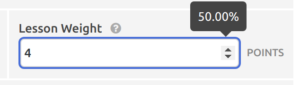Percentages are difficult to work with in this context because whenever the percentage value of one item is adjusted, all other items must also be simultaneously updated so that the total percentage value of all items always equals 100%. By using a weight instead of a percentage the total weights do not need to add up to 100.

For example the total available points in a quiz might be 8 (eight). Assuming there’s eight questions, each worth one point, each question would be worth 12.5% of the total grade of the quiz.

Should you choose to change the value of the last question of the quiz you can simply increase the number of points that question is worth until it reaches your desired percentage and you do not need to make any adjustments to any of the other 7 questions!

### Lesson Weighting

# Top

The Weight of a lesson represents the lesson’s percentage value within its course.

By default, all lessons are valued equally, each having a default Weight of 1 (one). A lesson with a higher Weight has greater impact on the overall grade of a course than a lesson with a lower Weight.

A common example would be a “Final Exam” which counts as a higher percentage of the overall course grade than the other “regular” quizzes.

To create a course with this structure you could configure the lesson Weight values as follows;

```Lesson 1 - Weight 1 (12.5%)
Lesson 2 - Weight 1 (12.5%)
Lesson 3 - Weight 1 (12.5%)
Lesson 4 - Weight 1 (12.5%)
Lesson 5 - Weight 4 (50%)```

If the lesson contains no quiz or assignment it automatically has a Weight of 0 (zero) and is excluded for grading calculations automatically.

The Weight of a lesson is set within the lesson settings in the Course Builder.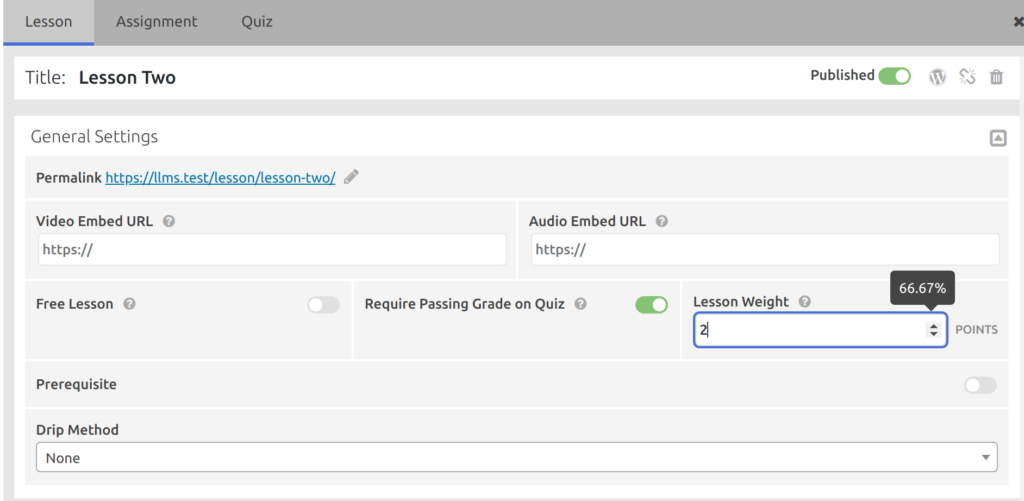The lesson Weight is entered into the text field and the percentage value is automatically calculated and displayed in a tooltip.

### Assignment and Quiz Weighting within a Lesson

# Top

When a lesson has both an assignment and a quiz, additional weighting options are made available to configure how each item impacts the calculated grade of the lesson.

By default, the Quiz Weight and Assignment Weight values are set to 1 (one). This means that the quiz and assignment each make up 50% of the lesson’s overall grade.

The Quiz Weight option is located on in the Quiz Settings area of the Course Builder: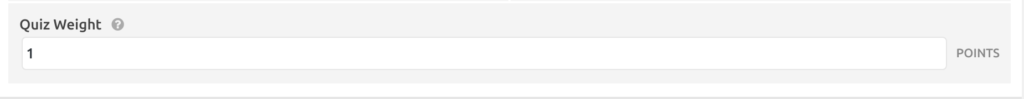The Assignment Weight option is located on in the Assignment Settings area of the Course Builder: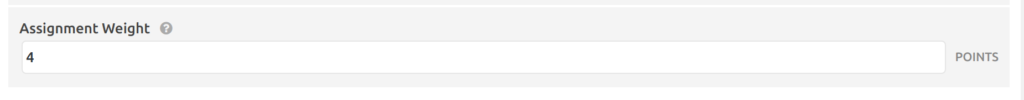### Quiz Question Weighting

# Top

Just as the Lesson Weight allows weighting of lessons within a course, Quiz Question Weights make it possible to change the values of questions within a quiz.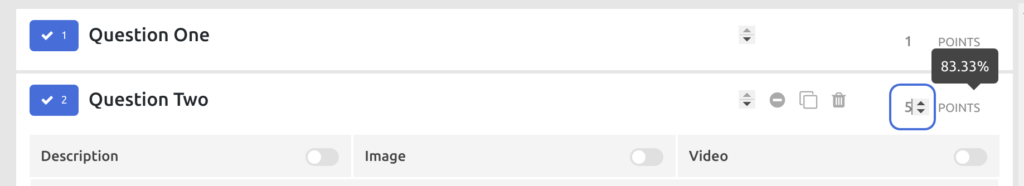### Excluding items with a Zero Weight

# Top

Any weighted item in LifterLMS can be completely excluded from the resulting grade by setting it’s Weight to 0 (zero).

This will work for Lesson Weight, Quiz Weight, Assignment Weight, or Question Points.

### Calculating the Grade of a Course

# Top

When calculating the overall grade of a course, LifterLMS takes the following steps:

1. Calculate the Total Available Points for the course
Add together all the individual Weight values for each lesson in the course
Multiply the Lesson Grade by the Lesson Weight for the lesson
4. Calculate the Overall Course Grade
Divide the Weight-Adjusted Total by the Total Available Points
##### Example
```Lesson    Weight  Percentage  Grade
Lesson A  1       12.5%       80%
Lesson B  1       12.5%       75%
Lesson C  1       12.5%       50%
Lesson D  1       12.5%       90%
Lesson E  4       50%         85%```

1. Total Available Points: 1 + 1 + 1 + 1 + 4 = 8

```Lesson    Weight  Percentage  Grade  Weight-Adjusted Grade
Lesson A  1       12.5%       80%    80 = 1 x 80
Lesson B  1       12.5%       75%    75 = 1 x 75
Lesson C  1       12.5%       50%    50 = 1 x 50
Lesson D  1       12.5%       90%    90 = 1 x 90
Lesson E  4       50%         85%    340 = 4 * 85```

3. Weight-Adjusted Total: 80 + 75 + 50 + 90 + 340 = 635
: 635 / 8 = 79.375%

### Calculating the Grade of a Lesson

# Top

There are four different scenarios for a lesson when determining its grade:

A. Lesson without a quiz or an assignment

In this scenario the lesson’s Weight is always 0 (zero) and it is excluded from the course grading calculations.

B. Lesson with a quiz only

In this scenario the lesson’s grade is equal to the quiz grade.

C. Lesson with an assignment only

In this scenario the lesson’s grade is equal to the assignment grade.

D. Lesson with a quiz and an assignment

In this scenario the grades of both the quiz and assignment are used to calculate the lesson grade.

1. Calculate the Total Available Points for the lesson
Add the Quiz Weight to the Assignment Weight
Multiply the Quiz Grade by the Quiz Weight
Multiply the Assignment Grade by the Assignment Weight
Divide Weight-Adjusted Total by the Total Available Points
##### Example
```Part       Weight  Percentage  Grade
Quiz       6       60%         70%
Assignment 4       40%         100%```

1. Total Available Points: 4 + 6 = 10
: 70 x 6 = 420
4. Weight-Adjusted Total: 420 + 160 = 580
5. Lesson Grade: 580 / 10 = 58%

### Calculating the Grade of a Quiz

# Top

A quiz’s grade is calculated similarly to the grade of a course except that questions do not receive their own individual percentage grades and are instead awarded a number of points. To calculate a lesson grade:

1. Calculate the Total Available Points for the quiz
Add together the individual Points value of each question in the quiz
2. Calculate the Weight-Adjusted Points Value for each point in the quiz
Divide the Total Available Points by 100
3. Calculate the Earned Points for the quiz attempt
Add together the total of all points for each correctly answered quiz question.
Multiply the Earned Points by the Weight-Adjusted Points Value
##### Example
```Question    Points  Percentage  Earned
Question A  3       20%         3
Question B  3       20%         3
Question C  3       20%         0
Question D  6       40%         4```

1. Total Available Points: 3 + 3 + 3 + 6 = 15
2. Weight-Adjusted Points Value: 100 / 15 = 6.667
3. Earned Points:
3 + 3 + 0 + 4 = 10